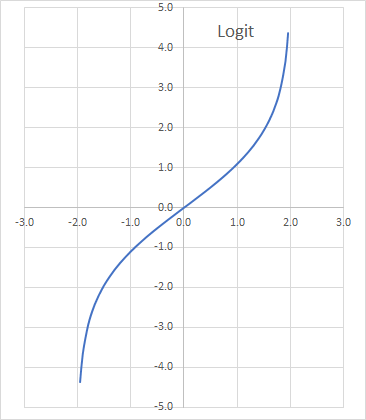# LOGIT - Logit Transform

Computes the logit transformation, including its inverse.

## Syntax

LOGIT(X, Lo, Hi, Return)

X
is the real value(s) for which we compute the transformation. It may be a single value or a one-dimensional array of cells (e.g., rows or columns)).
Lo
is the x-domain lower bound. If missing, Lo is assumed to be 0.
Hi
is the x-domain upper bound. If missing, Hi is assumed to be 1.
Return
is a number that determines the type of return value: 0 (or missing) = Logit, 1 = Inverse Logit.
Return Description
0 or omitted Logit Transform
1 Inverse of Logit transform

## Remarks

1. The X value(s) must be between Lo and Hi (exclusive).
2. The original Logit function is very commonly used for parameters that lie in the unit interval. Numerical values of theta close to 0 or 1 or out of range result in #VALUE! or #N/A.
3. The Logit transformation is defined as follows:
$$y=\textit{Logit}(x)=\ln{\frac{x}{1-x}}$$ And,
$$x=\textit{Logit}^{-1}(y)=\frac{e^y}{e^y+1}$$
Where:
• $x_{t}$ is the input value of the input time series at time $t$. X must be between 0 and 1, exclusive.
• $y_{t}$ is the transformed Logit value at time $t$.
• $\textit{Logit}^{-1}$ is the inverse Logit transformation.
4. To support a generic interval (Lo, Hi), we perform the following mapping:
$$z=\frac{x - Lo}{Hi - Lo}$$ So, the new transform and its inverse (i.e., $\textit{Logit}^{-1}$) are defined as follows:
$$y = ln{\frac{x - Lo}{Hi - x}}$$ $$x =\textit{Logit}^{-1}(y)= \frac{Lo+Hi\times{e^y}}{1+{e^y}}$$
5. In essence, the Logit converts a bounded x-range from (Lo, Hi) to $(-\infty, \infty)$:6. The Logit function accepts a single value or an array of values for X.
7. The first order derivative of the Logit transform is defined as follows:
$$\frac{dy}{dx}=\frac{Hi-Lo}{({x-Lo})({Hi-x})}$$
8. The first derivative is positive for all x-values in (Lo, Hi), but as x approaches Lo or Hi, the derivative goes to infinity.

## Examples

Example 1:

1
2
3
4
5
6
7
8
9
10
11
12
13
14
15
16
17
18
19
20
21
22
23
24
25
A B C D
Date Data LOGIT Inv-LOGIT
January 10, 2008 0.66 0.64 0.66
January 11, 2008 0.02 -3.99 0.02
January 12, 2008 0.54 0.18 0.54
January 13, 2008 0.21 -1.34 0.21
January 14, 2008 0.73 1.02 0.73
January 15, 2008 0.37 -0.52 0.37
January 16, 2008 1.00 6.25 1.00
January 17, 2008 0.42 -0.32 0.42
January 18, 2008 0.99 5.27 0.99
January 19, 2008 0.04 -3.22 0.04
January 20, 2008 0.23 -1.20 0.23
January 21, 2008 0.31 -0.79 0.31
January 22, 2008 0.69 0.82 0.69
January 23, 2008 0.37 -0.54 0.37
January 24, 2008 0.78 1.28 0.78
January 25, 2008 0.30 -0.86 0.30
January 26, 2008 0.97 3.45 0.97
January 27, 2008 0.91 2.29 0.91
January 28, 2008 0.92 2.40 0.92
January 29, 2008 0.88 1.97 0.88
January 30, 2008 0.14 -1.78 0.14
January 31, 2008 0.06 -2.81 0.06
February 1, 2008 0.19 -1.42 0.19
February 2, 2008 0.61 0.46 0.61